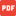1 . A right angle triangle in a semi-circle.pdf2. Two radii form an Isosceles Triangle.pdf3. Cyclic Quadrilateral.pdf4. The angles at the circumference made by in the same segment are equal.pdf